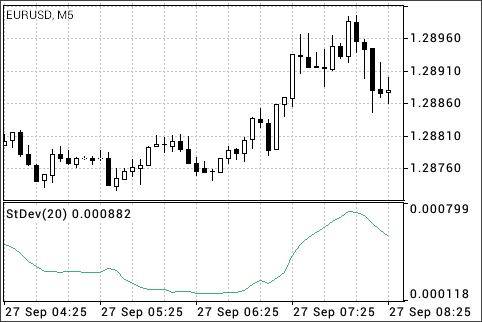MetaTrader 5 Android 帮助图表指标趋势指标标准偏差 (Standard Deviation)

标准偏差 (Standard Deviation)

• 如果其值太低，即，市场毫无生气，所以期待不久就会出现暴涨;
• 否则，如果其值极高，则意味着活力不久就会降温。计算公式:

StdDev (i) = SQRT (AMOUNT (j = i - N, i) / N)

AMOUNT (j = i - N, i) = SUM ((ApPRICE (j) - MA (ApPRICE , N, i)) ^ 2)

StdDev (i) ― 当前柱线的标准偏差;
SQRT ― 平方根;
AMOUNT(j = i - N, i) ― 从 j = i - N 至 i 的平方和;
N ― 平滑周期;
ApPRICE (j) ― 第 j 根柱线应用的价格;
MA (ApPRICE , N, i) ― 当前柱线上的 N 周期移动均值;
ApPRICE (i) ― 当前柱线的应用价格。